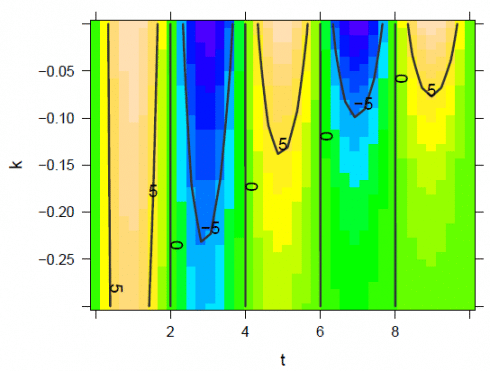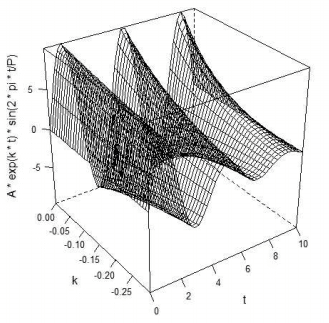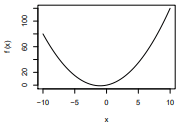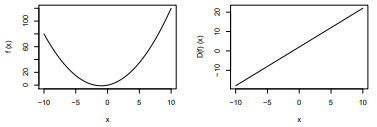# Calculus in R

June 27, 2019
By

Want to share your content on R-bloggers? click here if you have a blog, or here if you don't.

Are you interested in guest posting? Publish at DataScience+ via your editor (i.e., RStudio).

## Introdutcion to Calculus

Calculus is a branch of mathematics that involves the study of rates of change. Before calculus was invented, all math was static: It could only help calculate objects that were perfectly still. But the universe is constantly moving and changing. No objects—from the stars in space to subatomic particles or cells in the body—are always at rest. Indeed, just about everything in the universe is constantly moving. Calculus helped to determine how particles, stars, and matter actually move and change in real time.

Calculus is used in a multitude of fields that you wouldn’t ordinarily think would make use of its concepts. Among them are physics, engineering, economics, statistics, and medicine. Calculus is also used in such disparate areas as space travel, as well as determining how medications interact with the body, and even how to build safer structures. You’ll understand why calculus is useful in so many areas if you know a bit about its history as well as what it is designed to do and measure.

Math is one of the key building blocks of data science. If you want to understand what’s going on under the hood in your machine learning work as a data scientist, you’ll need to have a solid grasp of the fundamentals of calculus. While you cannot do a lot of data science with just calculus, the topic is essential for more advanced topics in data science such as machine learning, algorithms, and advanced statistics.

Let’s get started using calculus with R:

## Functions in R

Let us first try to do a function and

```library(mosaic)
f <- makeFun(m * x + b ~ x, m = 3.5, b = 10)
f(x = 2)
 17

```
```g <- makeFun(A * x * cos(pi * x * y) ~ x + y, A = 3)
g
function (x, y, A = 3)
A * x * cos(pi * x * y)
g(x = 1, y = 2)
 3

```

Let us try plotting the calculus function

```plotFun(A * exp(k * t) * sin(2 * pi * t/P) ~ t + k, t.lim = range(0, 10), k.lim = range(-0.3,0), A = 10, P = 4)
```

The function looks like this:Let us try do do another function in R:

```library(manipulate)
plotFun(A * exp(k * t) * sin(2 * pi * t/P) ~ t + k, t.lim = range(0, 10),k.lim = range(-0.3,0), A = 10, P = 4, surface = TRUE)
```

This function looks like this:Let us try do do a third function in R:

```plotFun(dt(t, df) ~ t + df, t.lim = range(-3,3), df.lim = range(1,10))
```

## Derivative Functions in R

Now let us try to do the derivative of a function in r:

```library(mosaic)
library(mosaicCalc)
D(sin(x) ~ x)
function (x)
cos(x)

```

Let us try to do a second derivative in R:

```D(A * x^2 * sin(y) ~ x)
function (x, A, y)
A * (2 * x) * sin(y)

```

Let us try to do a third derivative in R:

```D(A * x^2 * sin(y) ~ x + y)
function (x, y, A)
A * (2 * x) * cos(y)

```

## Solving in R

The findZeros() function will locate zeros of a function in a flexible way that’s easy to use. The
syntax is very similar to that of plotFun() and D().

Let us try solving in R:

```findZeros(sin(t) ~ t, t.lim = range(-5, 1))

```

Let us Find the nearest several zeros to a point:

```findZeros(sin(t) ~ t, nearest = 5, near = 10)

t
1 3.142
2 6.283
3 9.425
4 12.566
5 15.708

```

Let us Specify a range via a center and width and solve this:

```findZeros(sin(t) ~ t, near = 0, within = 8)
t
1 -9.425
2 -6.283
3 -3.142
4 0.000
5 3.142
6 6.283
7 9.425

```

Let us solve a solution to 4 sin(3x) = 2:

```solve(4 * sin(3 * x) == 2 ~ x, near = 0, within = 1)
x
1 0.1746
2 0.8726

```

Let us findZeros() for nonlinear functions:

```findZeros(x * y^2 - 8 ~ x & y, sin(x * y) - 0.5 ~ x & y)
x y
1 1.813e+01 -0.66430
2 1.679e+00 -2.18270
3 3.427e-02 15.27887
4 5.792e+00 1.17530
6 9.904e+00 0.89876
7 6.336e+01 -0.35532
9 2.263e+03 0.05945

```

Let us try findZeros() for another nonlinear function:

```findZeros(x * y^2 - 8 ~ x & y, sin(x * y) - 0.5 ~ x & y, near = c(x = 20, y = 0),
within = c(x = 5, y = 1))
Warning: the condition has length > 1 and only the first element will be used
x y
1 3.427e-02 15.278880
2 4.147e+00 -1.388989
3 8.567e-01 3.055775
4 2.586e+05 -0.005562
7 3.427e-02 15.278880
8 4.198e+01 -0.436539
9 3.427e-02 15.278870

```

Let us solve the zeros of the function x^2 + y^2 + z^2 − 10 in the x, y, z space:

```findZeros(x^2 + y^2 + z^2 - 10 ~ x & y & z, near = 0, within = 4)
x y z
1 1.305 -1.8665 2.1939
2 1.790 -1.1697 2.3296
3 1.861 -0.6357 2.4765
4 2.060 -1.2607 2.0415
5 2.242 -1.1766 1.8951
6 2.626 -0.6005 1.6567
7 2.687 -0.7627 1.4832
8 2.768 0.4391 1.4638
9 2.815 1.1881 0.8153
10 2.844 0.9829 0.9738

```

If we want to to show the surface nicely, we need lots of points. Let us try 1000 points that as a rule of thumb are requested:

```s1 <- findZeros(x^2 + y^2 + z^2 - 10 ~ x & y & z, near = 0, within = 10, nearest = 1000)
cloud(z ~ x + y, data = s1, pch = 19)
```

The above coding gives us the following result:## Plotting Derivative Functions in R

Let us now try plotting some functions before taking the derivative of the function.

```D = function(f,delta=.000001){
function(x){ (f(x+delta) - f(x-delta))/(2*delta)} }
f = function(x){ x^2 + 2*x }
plot(f, 0, 10)
```

the above coding gives us the following plot:Now let us try plotting a function and the derivative of the function:

```f = function(x){ x^2 + 2*x }
plot(f, 0, 10)
plot(D(f), 0, 10)
```

The above voding gives us the following two plots:Now let us try plotting the derivative of the derivative function:

```plot(D(D(f)), 0, 10)
```

The above coding gives us the following result:Related Post

R-bloggers.com offers daily e-mail updates about R news and tutorials about learning R and many other topics. Click here if you're looking to post or find an R/data-science job.
Want to share your content on R-bloggers? click here if you have a blog, or here if you don't.